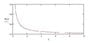# Internal Resistance of a Cell

• mayan
In summary, the internal resistance of a battery goes on increasing as the cell gets older because the battery is losing effectiveness due to the increased number of positive ions and reduced number of negative ions.

#### mayan

I want to understand that why does the internal resistance of a cell/battery goes on increasing as the cell gets older.

What happens inside the cell that causes the internal resistance to increase gradually?

Thanks.

I can't answer to the second question, but i can try to answer to the first:

Let's assume that you have a battery with some internal resistance $$R_{i}$$ and some $$R_{load} = 50\Omega$$ and they are coupled in series. Now, let's say that the battery voltage is constant and is $$V_{b} = 10V$$. Since you want to know what happens to the internal resistance we'll write a loop equation, that describes the circuit:

$$V_{b} = I\cdot (R_{i}+R_{load})$$

Re-writing the equation such that the current is a function of resistance gives:

$$R_{i}(I) = \frac{V_{b}}{I} - R_{load}$$, now we can plot the function

Looking at the function you can easily see that as the current drops, i.e you're using your battery for a long time, the internal resistance will increase.
Hence the result: Resistance will increase when the current drops, that could be found by using ohm's law, but i just wanted to show a more practical example.

P.S Y-axes is at - since the function by itself goes to infinity pretty quickly, so I had to go below zero to show the effect.

#### Attachments

•current.jpg
6.6 KB · Views: 605
Last edited:
Well, i know this thread is really old, but anyway, that question is more one based in chemistry rather than physics. I can't answer that for dry cell batteries, because I am not 100% sure how they work, but i can answer that for galvanic cells.

So first you have to understand how they work, galvanic cells have two different metals with different reduction potentials (meaning one has a higher affinity for electrons than the other) and they are placed in electrolytic liquids (usually just water with positive and negative ions floating around in them), so that the electrons can move between the two metals and they are also connected by a conducting wire (out of the water).

These galvanic cells are examples of redox reactions, so one of the metals is being reduced and the other is being oxidised. So one of the metals is losing electrons the other is gaining electrons. So the cathode, or the electrode/piece of metal at which reduction occurs, begins to get heavier as positive ions join with it/coat it and the Anode, at which oxidation occurs, loses mass as the cathode takes the elctrons from it.

The positive and negative ions floating around in the elctrolytic solutions, flow towards the electrode with the opposite charge to them (as opposites attract). Therefore all of the positive ions eventually end up at one electrode and all of the negative ions end up at the other.

The fact that one of the electrodes loses mass, combined with the fact that all of the charged particles end up where they want to be so they can no longer carry charge, and the fact that the cathode gets coated with the positive ions of the metal that the anode is made out of all contribute to reduced effectiveness in the battery.

## What is internal resistance of a cell?

Internal resistance of a cell is the opposition to the flow of electric current within the cell. It is caused by the resistance of the cell's components, such as the electrolyte solution and the electrodes.

## How is internal resistance measured?

Internal resistance can be measured by connecting a known external resistance to the cell and measuring the voltage across it. By using Ohm's law (R=V/I), the internal resistance of the cell can be calculated.

## What factors affect the internal resistance of a cell?

The internal resistance of a cell can be affected by various factors such as the type of electrolyte used, the size and material of the electrodes, and the temperature of the cell.

## How does internal resistance impact the performance of a cell?

Internal resistance can cause a voltage drop, which results in a reduction of the cell's voltage output. This can affect the cell's ability to deliver current and can also lead to a decrease in the cell's overall efficiency.

## Can the internal resistance of a cell be reduced?

While internal resistance is an inherent property of a cell, it can be reduced by using materials with lower resistance for the electrodes and choosing an appropriate electrolyte. Keeping the cell at a stable temperature and avoiding overcharging can also help reduce internal resistance.